# Fisseha Berhane, PhD

#### Data Scientist443-970-2353[email protected] CV Resume## Introduction to Spark programming¶

### Part 1. Quick overview

Spark is up to 100 times faster than Hadoop for certain applications and it is well suited to machine learning algorithms. One can read about Spark from spark website. Wikipedia also has general information about it.

Here, I am using the Python programming interface to Spark (pySpark which provides an easy-to-use programming abstraction and parallel runtime

Spark uses SparkContext to create RDDs

There are two types of operations. These are transformations and actions. Transformations are not computed immediately (they are lazy) e.g, map, filter. Transformed RDD is executed when action, such as collect and count, runs on it. We can also use cache to speed up operations.

Let's see a first example that adds the factorial of the first "n" non-negative integers. Let's use the xrange() function to create a list() of integers. xrange() only generates values as they are needed. This is different from the behavior of range() which generates the complete list upon execution. Because of this xrange() is more memory efficient than range(), especially for large ranges.

In :
fish = xrange(101) # considering 0 to 100 inclusive

In :
# Parallelize data using 8 partitions
# This operation is a transformation of data into an RDD
# Spark uses lazy evaluation, so no Spark jobs are ran at this point

factRDD = sc.parallelize(fish, 8)


Now, let us write a simple python function that computes the factorial of a number and squares it.

In :
import math

In :
# create a function that takes a number and calculates its factorial and squares it.
def myfunc(x):
return (math.factorial(x)**2)

In :
# Transform xrangeRDD through map transformation using myfunc function
# Because map is a transformation and Spark uses lazy evaluation, no jobs, stages,
# or tasks will be launched when we run this code.
factRDD1 = factRDD.map(myfunc)

In :
# Obtain Python's add function
from operator import add

In :
# Let's add and get the data

8710653556213906593217910546751236826770075534889462661969685875552902356740023437770774407535725726293284584188324726120379196905024071726217538933934155513097657108629996497860433734881084395513260664638775792257178708682117770296229335023994961148002046177649327941362944395975863609110502747608704759517069851818


Simple enough!

We can use the function filter() to get values that fulfill certain criteria.

In :
fish = xrange(101) # considering 0 to 100 inclusive

In :
# Parallelize data using 8 partitions
# This operation is a transformation of data into an RDD
# Spark uses lazy evaluation, so no Spark jobs are ran at this point

myRDD = sc.parallelize(fish, 8)

In :
# Let's get numbers divisible by 7
myRDDdiv7 = myRDD.filter(lambda x:x%7==0)

In :
# Let's collect the data
print myRDDdiv7.collect()

[0, 7, 14, 21, 28, 35, 42, 49, 56, 63, 70, 77, 84, 91, 98]


Let's add the first 10,000 non-negative integers

In :
fish = xrange(10001) # considering 0 to 10000 inclusive

In :
# Parallelize data using 8 partitions
# This operation is a transformation of data into an RDD
# Spark uses lazy evaluation, so no Spark jobs are ran at this point

myRDD = sc.parallelize(fish, 8)

In :
# add the numbers

50005000


### Part 2: Spark Context

#### The diagram below shows an example cluster, where the cores allocated for an application are outlined in purple.¶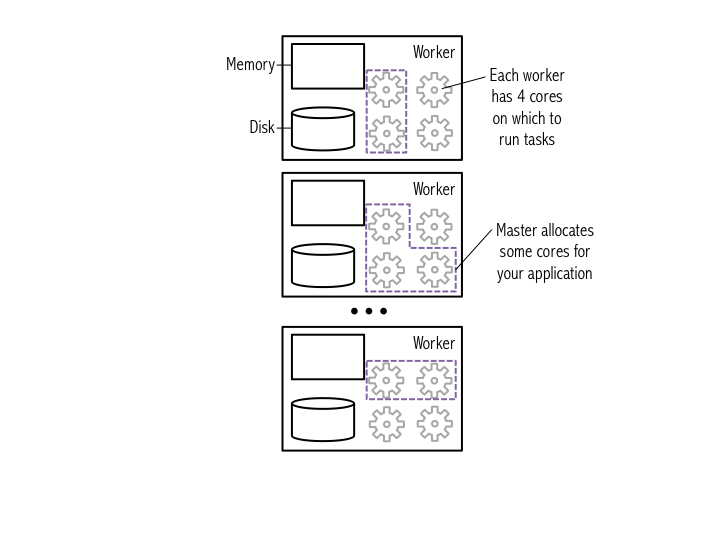#### Try printing out sc to see its type.¶

In :
# Display the type of the Spark Context sc
type(sc)

Out:
pyspark.context.SparkContext

#### You can use Python's dir() function to get a list of all the attributes (including methods) accessible through the sc object.¶

In :
# List sc's attributes
dir(sc)


#### Alternatively, you can use Python's help() function to get an easier to read list of all the attributes, including examples, that the sc object has.¶

In :
# Use help to obtain more detailed information
help(sc)

In :
# After reading the help we've decided we want to use sc.version to see what version of Spark we are running
sc.version

Out:
u'1.3.1'
In :
# Help can be used on any Python object
help(map)

Help on built-in function map in module __builtin__:

map(...)
map(function, sequence[, sequence, ...]) -> list

Return a list of the results of applying the function to the items of
the argument sequence(s).  If more than one sequence is given, the
function is called with an argument list consisting of the corresponding
item of each sequence, substituting None for missing values when not all
sequences have the same length.  If the function is None, return a list of
the items of the sequence (or a list of tuples if more than one sequence).



### Part 3: Using RDDs and chaining together transformations and actions¶

#### We will perform several exercises to obtain a better understanding of RDDs:¶

• ##### Create a Python collection of 10,000 integers
• ##### Create a Spark base RDD from that collection
• ##### Subtract one from each value using map
• ##### Perform action collect to view results
• ##### Perform action count to view counts
• ##### Apply transformation filter and view results with collect
• ##### Learn about lambda functions
• ##### Explore how lazy evaluation works and the debugging challenges that it introduces

#### We will use the xrange() function to create a list() of integers. xrange() only generates values as they are needed. This is different from the behavior of range() which generates the complete list upon execution. Because of this xrange() is more memory efficient than range(), especially for large ranges.¶

In :
data = xrange(1, 10001)

In :
type(data)

Out:
xrange
In :
# Data is just a normal Python list
# Obtain data's first element
data

Out:
1
In :
data

Out:
101
In :
# We can check the size of the list using the len() function
len(data)

Out:
10000

#### The figure below illustrates how Spark breaks a list of data entries into partitions that are each stored in memory on a worker.¶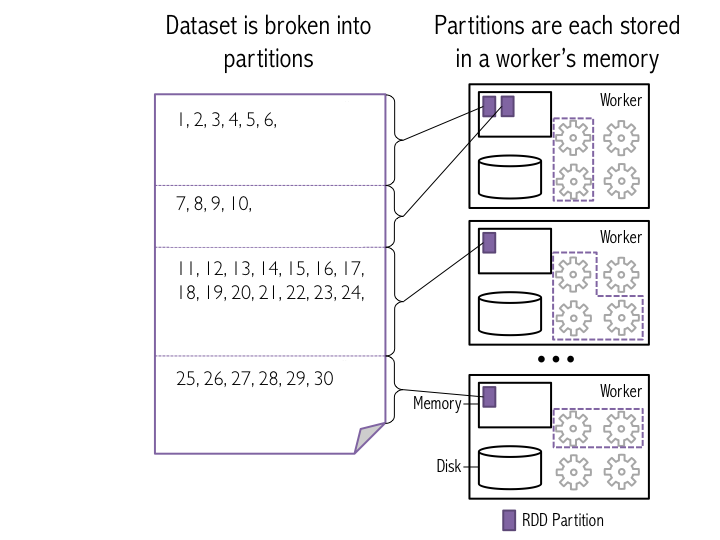#### After we generate RDDs, we can view them in the "Storage" tab of the web UI. You'll notice that new datasets are not listed until Spark needs to return a result due to an action being executed. This feature of Spark is called "lazy evaluation". This allows Spark to avoid performing unnecessary calculations.¶

In :
# Parallelize data using 8 partitions
# This operation is a transformation of data into an RDD
# Spark uses lazy evaluation, so no Spark jobs are run at this point
xrangeRDD = sc.parallelize(data, 8)

In :
# Let's view help on parallelize
help(sc.parallelize)

Help on method parallelize in module pyspark.context:

parallelize(self, c, numSlices=None) method of pyspark.context.SparkContext instance
Distribute a local Python collection to form an RDD. Using xrange
is recommended if the input represents a range for performance.

>>> sc.parallelize([0, 2, 3, 4, 6], 5).glom().collect()
[, , , , ]
>>> sc.parallelize(xrange(0, 6, 2), 5).glom().collect()
[[], , [], , ]


In :
# Let's see what type sc.parallelize() returned
print 'type of xrangeRDD: {0}'.format(type(xrangeRDD))

# How about if we use a range
dataRange = range(1, 10001)
rangeRDD = sc.parallelize(dataRange, 8)
print 'type of dataRangeRDD: {0}'.format(type(rangeRDD))

type of xrangeRDD: <class 'pyspark.rdd.PipelinedRDD'>
type of dataRangeRDD: <class 'pyspark.rdd.RDD'>

In :
# Each RDD gets a unique ID
print 'xrangeRDD id: {0}'.format(xrangeRDD.id())
print 'rangeRDD id: {0}'.format(rangeRDD.id())

xrangeRDD id: 76
rangeRDD id: 75

In :
# We can name each newly created RDD using the setName() method
xrangeRDD.setName('My first RDD')

Out:
My first RDD PythonRDD at RDD at PythonRDD.scala:43
In :
# Let's view the lineage (the set of transformations) of the RDD using toDebugString()
print xrangeRDD.toDebugString()

(8) My first RDD PythonRDD at RDD at PythonRDD.scala:43 []
|  ParallelCollectionRDD at parallelize at PythonRDD.scala:392 []

In :
# Let's use help to see what methods we can call on this RDD
help(xrangeRDD)

In :
# Let's see how many partitions the RDD will be split into by using the getNumPartitions()
xrangeRDD.getNumPartitions()

Out:
8

#### map(f), the most common Spark transformation, is one such example: it applies a function f to each item in the dataset, and outputs the resulting dataset. When you run map() on a dataset, a single stage of tasks is launched. A stage is a group of tasks that all perform the same computation, but on different input data. One task is launched for each partitition, as shown in the example below. A task is a unit of execution that runs on a single machine. When we run map(f) within a partition, a new task applies f to all of the entries in a particular partition, and outputs a new partition. In this example figure, the dataset is broken into four partitions, so four map() tasks are launched.¶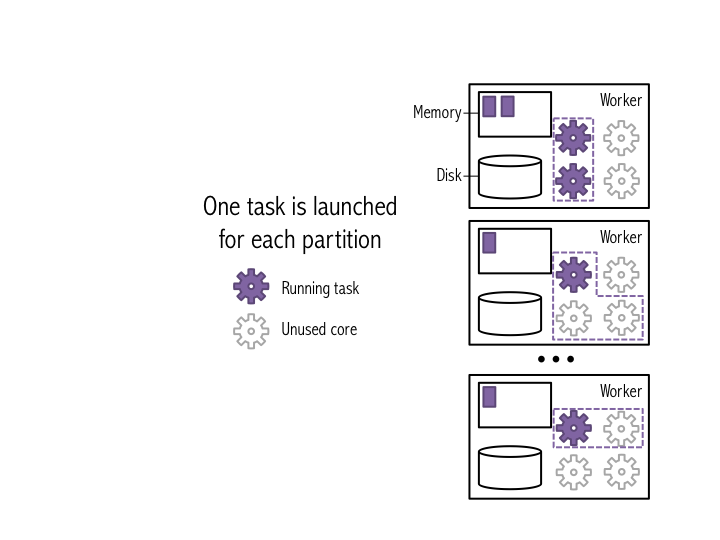#### The figure below shows how this would work on the smaller data set from the earlier figures. Note that one task is launched for each partition.¶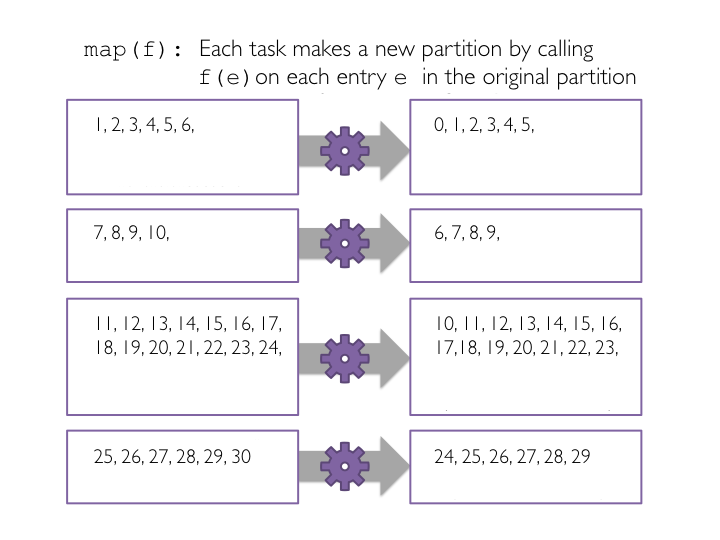#### Now we will use map() to subtract one from each value in the base RDD we just created. First, we define a Python function called sub() that will subtract one from the input integer. Second, we will pass each item in the base RDD into a map() transformation that applies the sub() function to each element. And finally, we print out the RDD transformation hierarchy using toDebugString().¶

In :
# Create sub function to subtract 1
def sub(value):
""""Subtracts one from value.

Args:
value (int): A number.

Returns:
int: value minus one.
"""
return (value - 1)

# Transform xrangeRDD through map transformation using sub function
# Because map is a transformation and Spark uses lazy evaluation, no jobs, stages,
# or tasks will be launched when we run this code.
subRDD = xrangeRDD.map(sub)

# Let's see the RDD transformation hierarchy
print subRDD.toDebugString()

(8) PythonRDD at RDD at PythonRDD.scala:43 []
|  ParallelCollectionRDD at parallelize at PythonRDD.scala:392 []


#### In this example, the dataset is broken into four partitions, so four collect() tasks are launched. Each task collects the entries in its partition and sends the result to the SparkContext, which creates a list of the values, as shown in the figure below.¶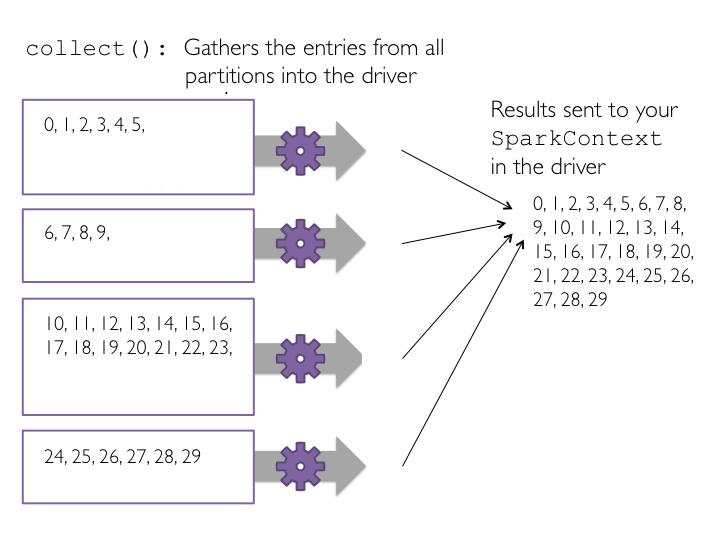#### Now let's run collect() on subRDD.¶

In :
# Let's collect the data
print subRDD.collect()


#### Each task counts the entries in its partition and sends the result to your SparkContext, which adds up all of the counts. The figure below shows what would happen if we ran count() on a small example dataset with just four partitions.¶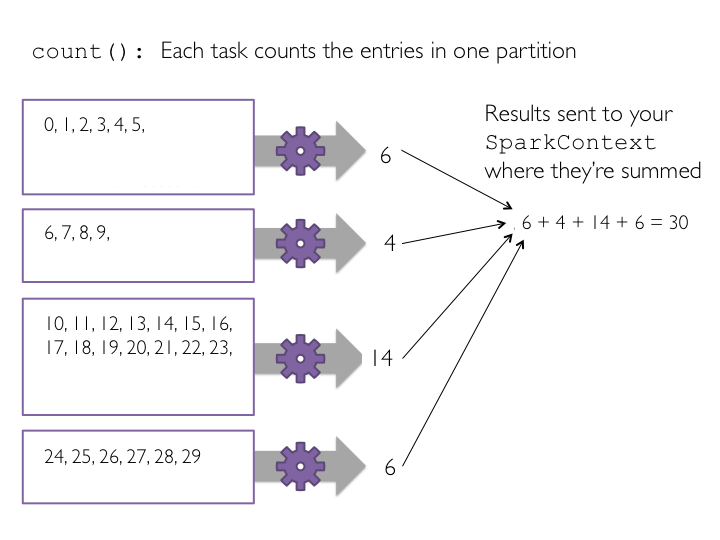In :
print xrangeRDD.count()
print subRDD.count()

10000
10000


#### The figure below shows how this would work on the small four-partition dataset.¶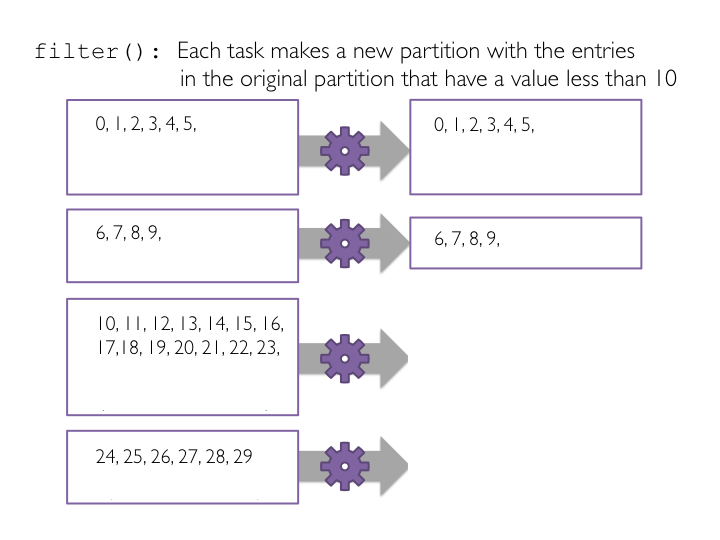#### To view the filtered list of elements less than ten, we need to create a new list on the driver from the distributed data on the executor nodes. We use the collect() method to return a list that contains all of the elements in this filtered RDD to the driver program.¶

In :
# Define a function to filter a single value
def ten(value):
"""Return whether value is below ten.

Args:
value (int): A number.

Returns:
bool: Whether value is less than ten.
"""
if (value < 10):
return True
else:
return False
# The ten function could also be written concisely as: def ten(value): return value < 10

# Pass the function ten to the filter transformation
# Filter is a transformation so no tasks are run
filteredRDD = subRDD.filter(ten)

# View the results using collect()
# Collect is an action and triggers the filter transformation to run
print filteredRDD.collect()

[0, 1, 2, 3, 4, 5, 6, 7, 8, 9]


### Part 4: Lambda Functions ¶

#### Here, instead of defining a separate function for the filter() transformation, we will use an inline lambda() function.¶

In :
lambdaRDD = subRDD.filter(lambda x: x < 10)
lambdaRDD.collect()

Out:
[0, 1, 2, 3, 4, 5, 6, 7, 8, 9]
In :
# Let's collect the even values less than 10
evenRDD = lambdaRDD.filter(lambda x: x % 2 == 0)
evenRDD.collect()

Out:
[0, 2, 4, 6, 8]

### Part 5: Additional RDD actions ¶

#### The reduce() action reduces the elements of a RDD to a single value by applying a function that takes two parameters and returns a single value. The function should be commutative and associative, as reduce() is applied at the partition level and then again to aggregate results from partitions. If these rules don't hold, the results from reduce() will be inconsistent. Reducing locally at partitions makes reduce() very efficient.¶

In :
# Let's get the first element
print filteredRDD.first()
# The first 4
print filteredRDD.take(4)
# Note that it is ok to take more elements than the RDD has
print filteredRDD.take(12)

0
[0, 1, 2, 3]
[0, 1, 2, 3, 4, 5, 6, 7, 8, 9]

In :
# Retrieve the three smallest elements
print filteredRDD.takeOrdered(3)
# Retrieve the five largest elements
print filteredRDD.top(5)

[0, 1, 2]
[9, 8, 7, 6, 5]

In :
# Pass a lambda function to takeOrdered to reverse the order
filteredRDD.takeOrdered(4, lambda s: -s)

Out:
[9, 8, 7, 6]
In :
# Obtain Python's add function
from operator import add
# Efficiently sum the RDD using reduce
# Sum using reduce with a lambda function
print filteredRDD.reduce(lambda a, b: a + b)
# Note that subtraction is not both associative and commutative
print filteredRDD.reduce(lambda a, b: a - b)
print filteredRDD.repartition(4).reduce(lambda a, b: a - b)
# While addition is
print filteredRDD.repartition(4).reduce(lambda a, b: a + b)

45
45
-45
21
45


#### The countByValue() action returns the count of each unique value in the RDD as a dictionary that maps values to counts.¶

In :
# takeSample reusing elements
print filteredRDD.takeSample(withReplacement=True, num=6)
# takeSample without reuse
print filteredRDD.takeSample(withReplacement=False, num=6)

[2, 9, 7, 5, 9, 0]
[6, 9, 8, 2, 5, 4]

In :
# Set seed for predictability
print filteredRDD.takeSample(withReplacement=False, num=6, seed=500)
# Try reruning this cell and the cell above -- the results from this cell will remain constant
# Use ctrl-enter to run without moving to the next cell

[0, 2, 5, 3, 6, 9]

In :
# Create new base RDD to show countByValue
repetitiveRDD = sc.parallelize([1, 2, 3, 1, 2, 3, 1, 2, 1, 2, 3, 3, 3, 4, 5, 4, 6])
print repetitiveRDD.countByValue()

defaultdict(<type 'int'>, {1: 4, 2: 4, 3: 5, 4: 2, 5: 1, 6: 1})


### Part 6: Additional RDD transformations ¶

#### To demonstrate flatMap(), we will first emit a word along with its plural, and then a range that grows in length with each subsequent operation.¶

In :
# Let's create a new base RDD to work from
wordsList = ['cat', 'elephant', 'rat', 'rat', 'cat']
wordsRDD = sc.parallelize(wordsList, 4)

# Use map
singularAndPluralWordsRDDMap = wordsRDD.map(lambda x: (x, x + 's'))
# Use flatMap
singularAndPluralWordsRDD = wordsRDD.flatMap(lambda x: (x, x + 's'))

# View the results
print singularAndPluralWordsRDDMap.collect()
print singularAndPluralWordsRDD.collect()
# View the number of elements in the RDD
print singularAndPluralWordsRDDMap.count()
print singularAndPluralWordsRDD.count()

[('cat', 'cats'), ('elephant', 'elephants'), ('rat', 'rats'), ('rat', 'rats'), ('cat', 'cats')]
['cat', 'cats', 'elephant', 'elephants', 'rat', 'rats', 'rat', 'rats', 'cat', 'cats']
5
10

In :
simpleRDD = sc.parallelize([2, 3, 4])
print simpleRDD.map(lambda x: range(1, x)).collect()
print simpleRDD.flatMap(lambda x: range(1, x)).collect()

[, [1, 2], [1, 2, 3]]
[1, 1, 2, 1, 2, 3]


#### Look at the diagram below to understand how reduceByKey works. Notice how pairs on the same machine with the same key are combined (by using the lamdba function passed into reduceByKey) before the data is shuffled. Then the lamdba function is called again to reduce all the values from each partition to produce one final result.¶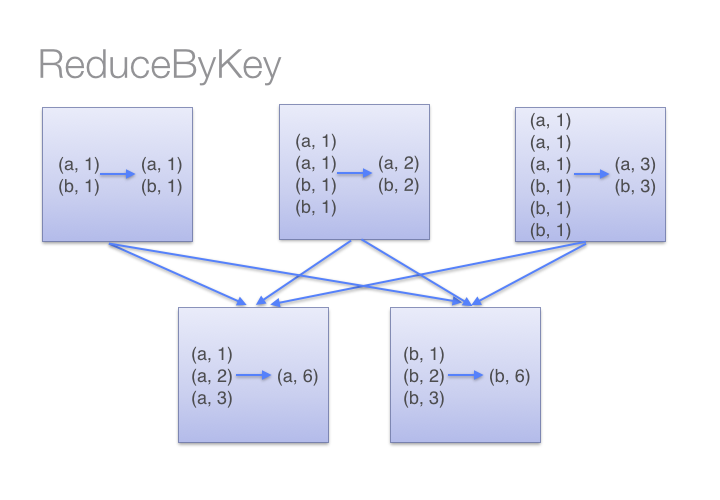#### To determine which machine to shuffle a pair to, Spark calls a partitioning function on the key of the pair. Spark spills data to disk when there is more data shuffled onto a single executor machine than can fit in memory. However, it flushes out the data to disk one key at a time, so if a single key has more key-value pairs than can fit in memory an out of memory exception occurs. This will be more gracefully handled in a later release of Spark so that the job can still proceed, but should still be avoided. When Spark needs to spill to disk, performance is severely impacted.¶#### Here are more transformations to prefer over groupByKey():¶

• #### combineByKey() can be used when you are combining elements but your return type differs from your input value type.
• #### foldByKey() merges the values for each key using an associative function and a neutral "zero value". #### Now let's go through a simple groupByKey() and reduceByKey() example.
In :
pairRDD = sc.parallelize([('a', 1), ('a', 2), ('b', 1)])
# mapValues only used to improve format for printing
print pairRDD.groupByKey().mapValues(lambda x: list(x)).collect()

# Different ways to sum by key
print pairRDD.groupByKey().map(lambda (k, v): (k, sum(v))).collect()
# Using mapValues, which is recommended when they key doesn't change
print pairRDD.groupByKey().mapValues(lambda x: sum(x)).collect()
# reduceByKey is more efficient / scalable

[('a', [1, 2]), ('b', )]
[('a', 3), ('b', 1)]
[('a', 3), ('b', 1)]
[('a', 3), ('b', 1)]


#### The mapPartitionsWithIndex() transformation uses a function that takes in a partition index (think of this like the partition number) and an iterator (to the items in that specific partition). For every partition (index, iterator) pair, the function returns a tuple of the same partition index number and an iterator of the transformed items in that partition.¶

In :
# mapPartitions takes a function that takes an iterator and returns an iterator
print wordsRDD.collect()
itemsRDD = wordsRDD.mapPartitions(lambda iterator: [','.join(iterator)])
print itemsRDD.collect()

['cat', 'elephant', 'rat', 'rat', 'cat']
['cat', 'elephant', 'rat', 'rat,cat']

In :
itemsByPartRDD = wordsRDD.mapPartitionsWithIndex(lambda index, iterator: [(index, list(iterator))])
# We can see that three of the (partitions) workers have one element and the fourth worker has two
# elements, although things may not bode well for the rat...
print itemsByPartRDD.collect()
# Rerun without returning a list (acts more like flatMap)
itemsByPartRDD = wordsRDD.mapPartitionsWithIndex(lambda index, iterator: (index, list(iterator)))
print itemsByPartRDD.collect()

[(0, ['cat']), (1, ['elephant']), (2, ['rat']), (3, ['rat', 'cat'])]
[0, ['cat'], 1, ['elephant'], 2, ['rat'], 3, ['rat', 'cat']]


### Part 7: Caching RDDs and storage options ¶

#### You can check if an RDD is cached by using the is_cached attribute, and you can see your cached RDD in the "Storage" section of the Spark web UI. If you click on the RDD's name, you can see more information about where the RDD is stored.¶

In :
# Name the RDD
filteredRDD.setName('My Filtered RDD')
# Cache the RDD
filteredRDD.cache()
# Is it cached
print filteredRDD.is_cached

True


#### Advanced: Spark provides many more options for managing how RDDs are stored in memory or even saved to disk. You can explore the API for RDD's persist() operation using Python's help() command. The persist() operation, optionally, takes a pySpark StorageLevel object.¶

In :
# Note that toDebugString also provides storage information
print filteredRDD.toDebugString()

(8) My Filtered RDD PythonRDD at collect at <ipython-input-77-2e6525e1a0c2>:23 [Memory Serialized 1x Replicated]
|  ParallelCollectionRDD at parallelize at PythonRDD.scala:392 [Memory Serialized 1x Replicated]

In :
# If we are done with the RDD we can unpersist it so that its memory can be reclaimed
filteredRDD.unpersist()
# Storage level for a non cached RDD
print filteredRDD.getStorageLevel()
filteredRDD.cache()
# Storage level for a cached RDD
print filteredRDD.getStorageLevel()

Serialized 1x Replicated
Memory Serialized 1x Replicated


### Part 8: Debugging Spark applications and lazy evaluation¶

#### The filter() method will not be executed until an action operation is invoked on the RDD. We will perform an action by using the collect() method to return a list that contains all of the elements in this RDD.¶

In :
def brokenTen(value):
"""Incorrect implementation of the ten function.

Note:
The if statement checks an undefined variable val instead of value.

Args:
value (int): A number.

Returns:
bool: Whether value is less than ten.

Raises:
NameError: The function references val, which is not available in the local or global
namespace, so a NameError is raised.
"""
if (val < 10):
return True
else:
return False

brokenRDD = subRDD.filter(brokenTen)

In :
# Now we'll see the error
brokenRDD.collect()


#### Scroll through the output "Py4JJavaError Traceback (most recent call last)" part of the cell and first you will see that the line that generated the error is the collect() method line. There is nothing wrong with this line. However, it is an action and that caused other methods to be executed. Continue scrolling through the Traceback and you will see the following error line:¶

NameError: global name 'val' is not defined


#### As you are learning Spark, I recommend that you write your code in the form:¶

RDD.transformation1()
RDD.action1()
RDD.transformation2()
RDD.action2()


#### Once you become more experienced with Spark, you can write your code with the form:¶

RDD.transformation1().transformation2().action()


#### We can also use lambda() functions instead of separately defined functions when their use improves readability and conciseness.¶

In :
# Cleaner code through lambda use
subRDD.filter(lambda x: x < 10).collect()

Out:
[0, 1, 2, 3, 4, 5, 6, 7, 8, 9]
In :
# Even better by moving our chain of operators into a single line.
sc.parallelize(data).map(lambda y: y - 1).filter(lambda x: x < 10).collect()

Out:
[0, 1, 2, 3, 4, 5, 6, 7, 8, 9]

#### To make the expert coding style more readable, enclose the statement in parentheses and put each method, transformation, or action on a separate line.¶

In :
# Final version
(sc
.parallelize(data)
.map(lambda y: y - 1)
.filter(lambda x: x < 10)
.collect())

Out:
[0, 1, 2, 3, 4, 5, 6, 7, 8, 9]